### Home > A2C > Chapter Ch10 > Lesson 10.1.2 > Problem10-46

10-46.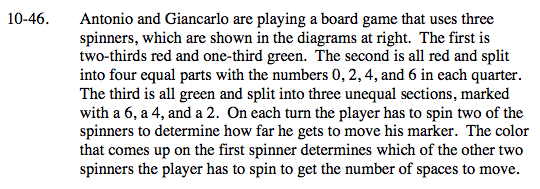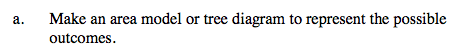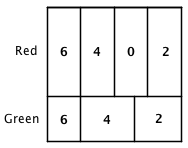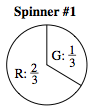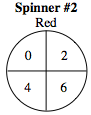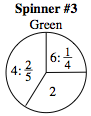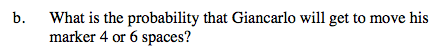Place probabilities on the appropriate sides and multiply to find the probabilities of the seven possible outcomes.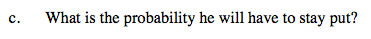$\frac{2}{3}\cdot\frac{1}{4}=\frac{1}{6}$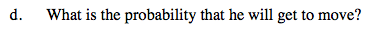$1-\frac{1}{6}=\frac{5}{6}$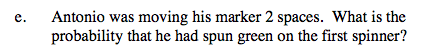Divide the probability of getting green and then 2 by the probability of getting 2 with either color.